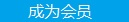您可以捐助，支持我们的公益事业。 1元 10元 50元 认证码：必填求知 文章 文库 Lib 视频 iProcess 课程 认证 咨询 工具 讲座 Modeler Code要资料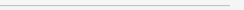订阅捐助
PyTorch可视化理解卷积神经网络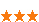643 次浏览     评价： 好 中 差
2019-1-31

 编辑推荐: 本文来自于segmentfault，文章使用图片加代码的形式讲解CNN网络，并对每层的输出进行可视化。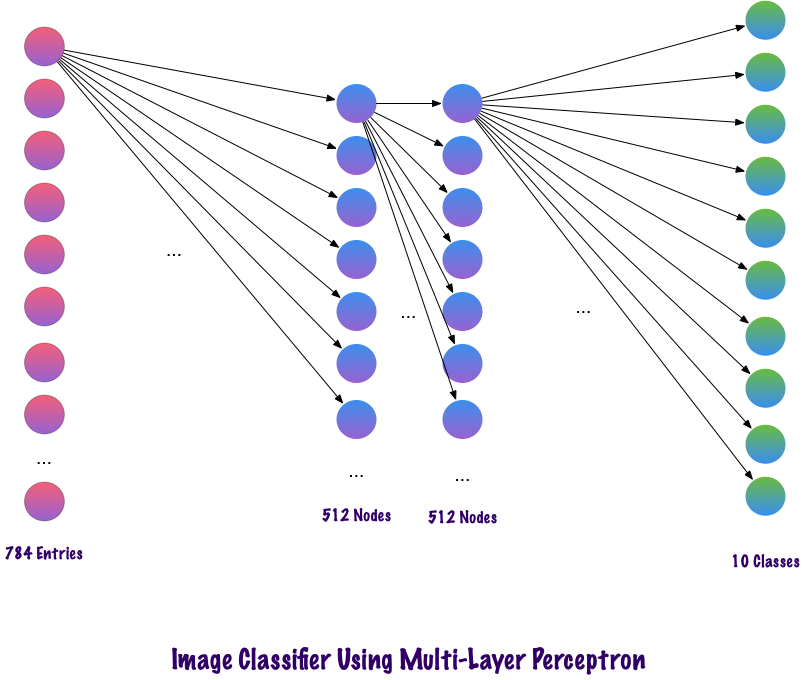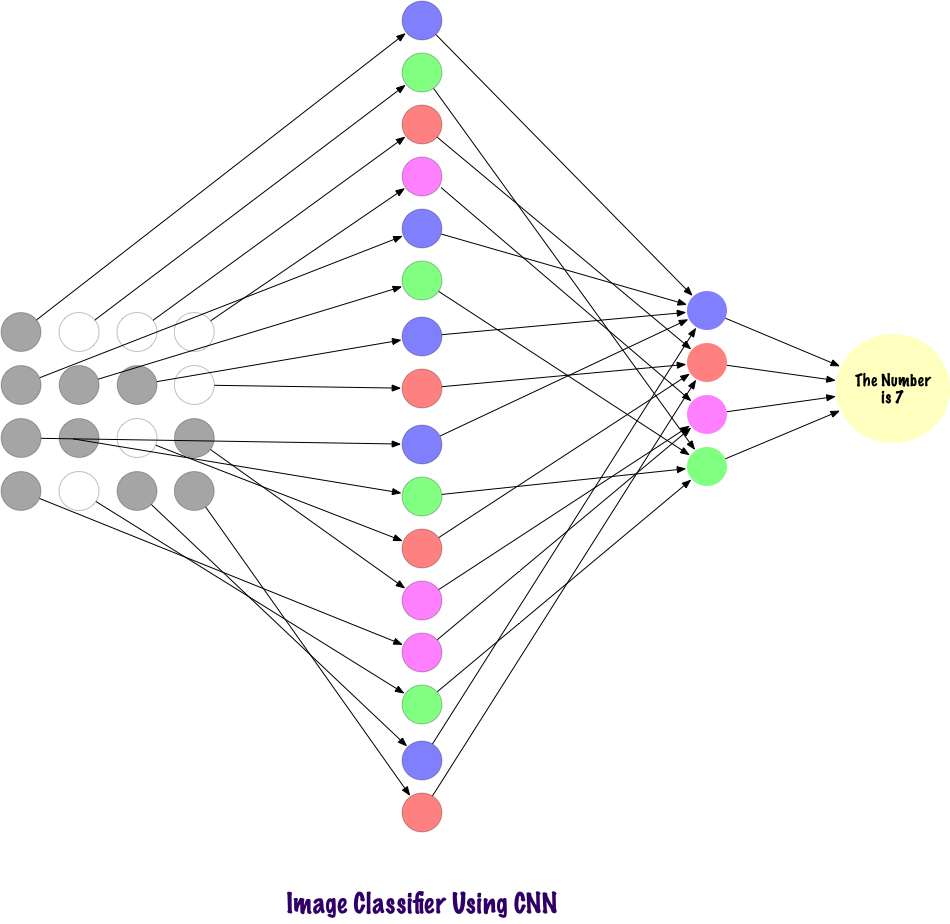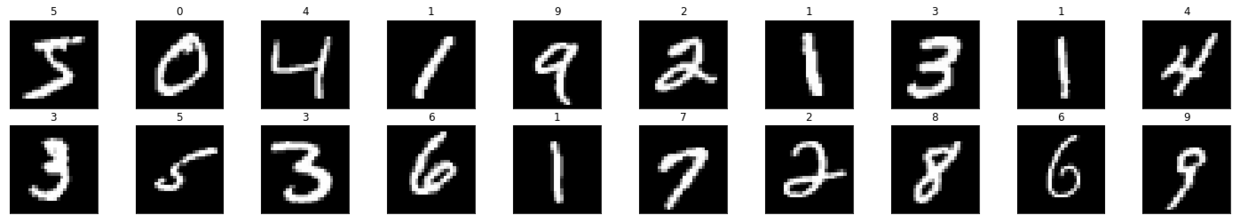img = np.squeeze(images) fig = plt.figure(figsize = (12,12)) ax = fig.add_subplot(111) ax.imshow(img, cmap='gray') width, height = img.shape thresh = img.max()/2.5 for x in range(width): for y in range(height): val = round(img[x][y],2) if img[x][y] !=0 else 0 ax.annotate(str(val), xy=(y,x), color='white' if img[x][y]

 Import matplotlib.pyplot as plt Import matplotib.image as mpimg Import cv2 Import numpy as np Image = mpimg.imread(‘dog.jpg’) Plt.imshow(image)# 转换为灰度图 gray = cv2.cvtColor(image, cv2.COLOR_RB2GRAY) # 定义sobel过滤器 sobel = np.array([-1, -2, -1], [0, 0, 0], [1, 2, 1])) # 应用sobel过滤器 Filtered_image = cv2.filter2D(gray, -1, sobel_y) # 画图 Plt.imshow(filtered_image, cmp=’gray’)1.卷积层（Convolutional layer）

2.池层(Pooling layer)

3.全连接层(fully connected layer)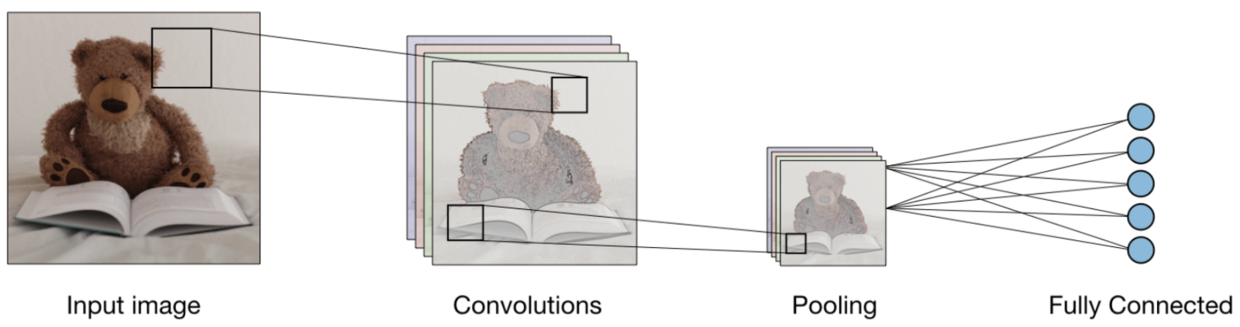* 卷积层（CONV）――使用过滤器执行卷积操作。因为它扫描输入图像的尺寸。它的超参数包括滤波器大小，可以是2x2、3x3、4x4、5x5（或其它）和步长S。结果输出O称为特征映射或激活映射，具有使用输入层计算的所有特征和过滤器。下面描绘了应用卷积的工作过程：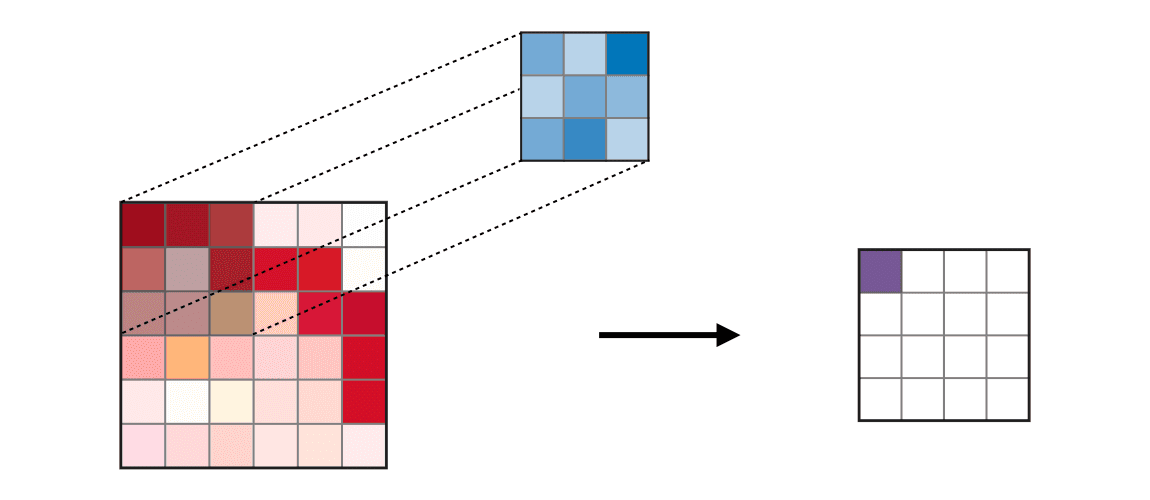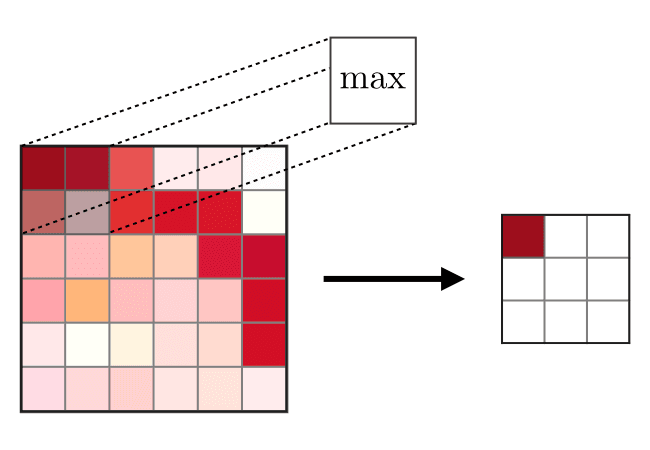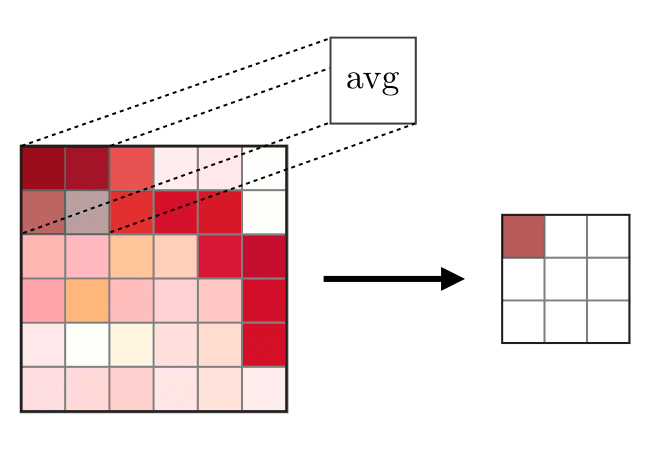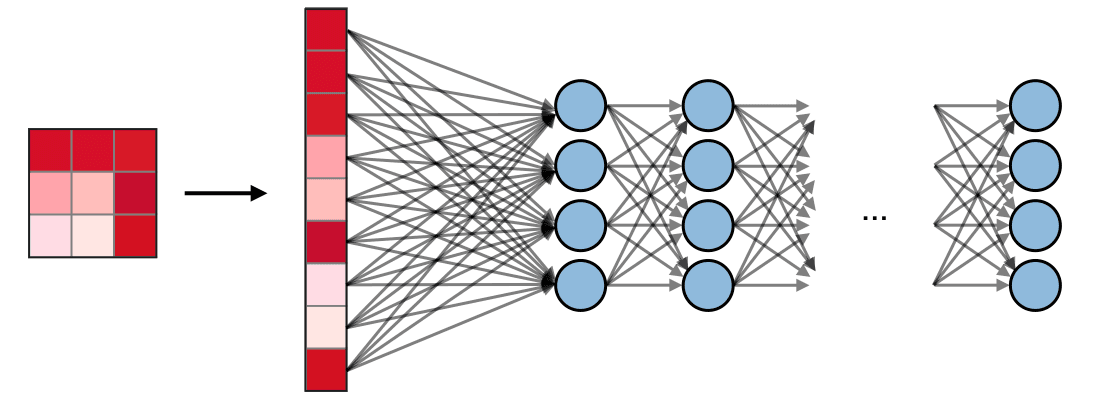import cv2 import matplotlib.pyplot as plt %matplotlib inline img_path = 'dog.jpg' bgr_img = cv2.imread(img_path) gray_img = cv2.cvtColor(bgr_img, cv2.COLOR_BGR2GRAY) # Normalise gray_img = gray_img.astype("float32")/255 plt.imshow(gray_img, cmap='gray') plt.show()import numpy as np filter_vals = np.array([ [-1, -1, 1, 1], [-1, -1, 1, 1], [-1, -1, 1, 1], [-1, -1, 1, 1] ]) print('Filter shape: ', filter_vals.shape) # Defining the Filters filter_1 = filter_vals filter_2 = -filter_1 filter_3 = filter_1.T filter_4 = -filter_3 filters = np.array([filter_1, filter_2, filter_3, filter_4]) # Check the Filters fig = plt.figure(figsize=(10, 5)) for i in range(4): ax = fig.add_subplot(1, 4, i+1, xticks=[], yticks=[]) ax.imshow(filters[i], cmap='gray') ax.set_title('Filter %s' % str(i+1)) width, height = filters[i].shape for x in range(width): for y in range(height): ax.annotate(str(filters[i][x][y]), xy=(y,x), color='white' if filters[i][x][y]<0 else 'black')

 import torch import torch.nn as nn import torch.nn.functional as F class Net(nn.Module): def __init__(self, weight): super(Net, self).__init__() # initializes the weights of the convolutional layer to be the weights of the 4 defined filters k_height, k_width = weight.shape[2:] # assumes there are 4 grayscale filters self.conv = nn.Conv2d(1, 4, kernel_size=(k_height, k_width), bias=False) # initializes the weights of the convolutional layer self.conv.weight = torch.nn.Parameter(weight) # define a pooling layer self.pool = nn.MaxPool2d(2, 2) def forward(self, x): # calculates the output of a convolutional layer # pre- and post-activation conv_x = self.conv(x) activated_x = F.relu(conv_x) # applies pooling layer pooled_x = self.pool(activated_x) # returns all layers return conv_x, activated_x, pooled_x # instantiate the model and set the weights weight = torch.from_numpy(filters).unsqueeze(1).type(torch.FloatTensor) model = Net(weight) # print out the layer in the network print(model)

 Net( (conv): Conv2d(1, 4, kernel_size=(4, 4), stride=(1, 1), bias=False) (pool): MaxPool2d(kernel_size=2, stride=2, padding=0, dilation=1, ceil_mode=False) )

 def viz_layer(layer, n_filters= 4): fig = plt.figure(figsize=(20, 20)) for i in range(n_filters): ax = fig.add_subplot(1, n_filters, i+1) ax.imshow(np.squeeze(layer[0,i].data.numpy()), cmap='gray') ax.set_title('Output %s' % str(i+1)) fig = plt.figure(figsize=(12, 6)) fig.subplots_adjust(left=0, right=1.5, bottom=0.8, top=1, hspace=0.05, wspace=0.05) for i in range(4): ax = fig.add_subplot(1, 4, i+1, xticks=[], yticks=[]) ax.imshow(filters[i], cmap='gray') ax.set_title('Filter %s' % str(i+1)) gray_img_tensor = torch.from_numpy(gray_img).unsqueeze(0).unsqueeze(1)643 次浏览  评价: 好 中 差订阅捐助
 相关文章 我们该如何设计数据库 数据库设计经验谈 数据库设计过程 数据库编程总结
 相关文档 数据库性能调优技巧 数据库性能调整 数据库性能优化讲座 数据库系统性能调优系列
 相关课程 高性能数据库设计与优化 高级数据库架构师 数据仓库和数据挖掘技术 Hadoop原理、部署与性能调优每天2个文档/视频 扫描微信二维码订阅
 订阅技术月刊 获得每月300个技术资源